Next: Graph Transformation Method Up: ENSEMBLES OF REARRANGEMENT PATHWAYS Previous: Chain Graphs   Contents

# Complete Graphs

In a complete digraph each pair of nodes is connected by two oppositely directed edges . The complete graph with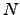graph nodes is denoted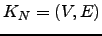, and hasnodes and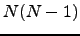edges, remembering that we have two edges per connection (Figure 4.4).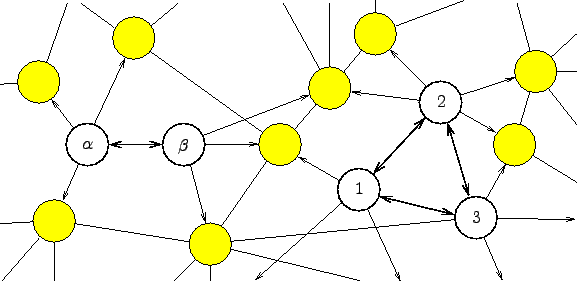Due to the complete connectivity we need only consider two cases: when the starting and finishing nodes are the same and when they are distinct. We employ complete graphs for the purposes of generality. An arbitrary graph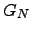is a subgraph of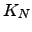with transition probabilities for non-existent edges set to zero. All the results in this section are therefore equally applicable to arbitrary graphs.

The complete graph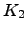will not be considered here as it is topologically identical to the graph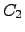. The difference between the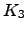and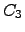graphs is the existence of edges that connect nodes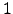and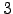. Pathways confined tocan therefore contain cycles, and for a given path length they are significantly more numerous (Figure 4.5).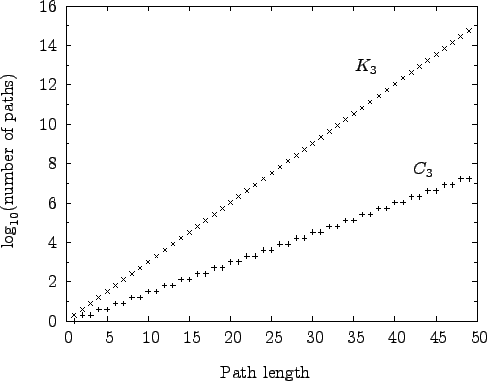The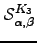can again be derived analytically for this graph: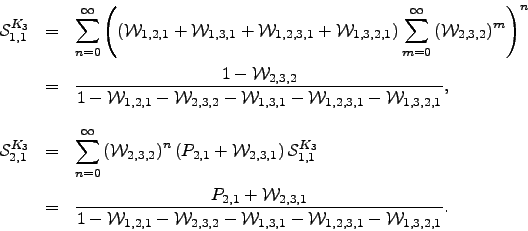(6.30)

The results for any other possibility can be obtained by permuting the node indices appropriately.

The pathway sums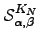for larger complete graphs can be obtained by recursion. For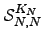any path leaving from and returning tocan be broken down into a step out ofto any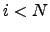, all possible paths between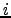and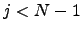within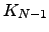, and finally a step back tofrom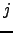. All such paths can be combined together in any order, so we have a multinomial distribution :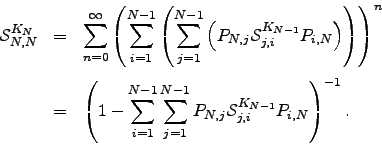(6.31)

To evaluate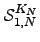we break down the sum into all paths that depart from and return to, followed by all paths that leave nodeand reach node 1 without returning to. The first contribution corresponds to a factor of, and the second produces a factor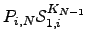for every: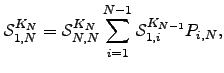(6.32)

where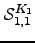is defined to be unity. Any othercan be obtained by a permutation of node labels.

Algorithm B.2 provides an example implementation of the above formulae optimised for incomplete graphs. The running time of Algorithm B.2 depends strongly on the graph density. (A digraph in which the number of edges is close to the maximum value ofis termed a dense digraph .) Forthe algorithm runs in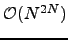time, while for an arbitrary graph it scales as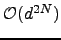, where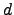is the average degree of the nodes. For chain graphs the algorithm runs in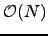time and therefore constitutes a recursive-function-based alternative to Algorithm B.1 with linear memory requirements. For complete graphs an alternative implementation with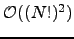scaling is also possible.

Although the scaling of the above algorithm withmay appear disastrous, it does in fact run faster than standard KMC and MM approaches for graphs where the escape probabilities are several orders of magnitude smaller than the transition probabilities (Algorithm B.2). Otherwise, for anything but moderately branched chain graphs, Algorithm B.2 is significantly more expensive. However, the graph-transformation-based method presented in Section 4.4 yields both the pathway sums and the mean escape times for a complete graphin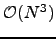time, and is the fastest approach that we have found.

Mean escape times forare readily obtained from the results in Equation 4.30 by applying the method outlined in Section 4.1.5:(6.33)

We have verified this result analytically using first-step analysis and numerically for various values of the parameters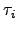and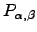. and obtained quantitative agreement (see Figure 4.6).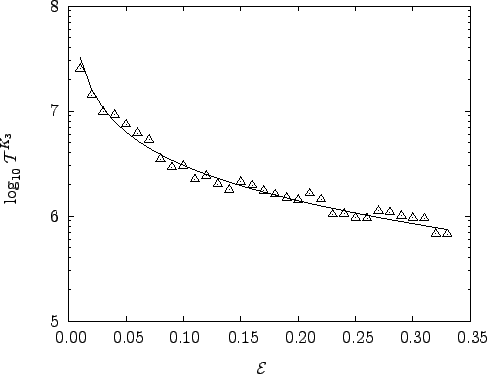Figure 4.7 demonstrates how the advantage of exact summation over KMC and MM becomes more pronounced as the escape probabilities become smaller.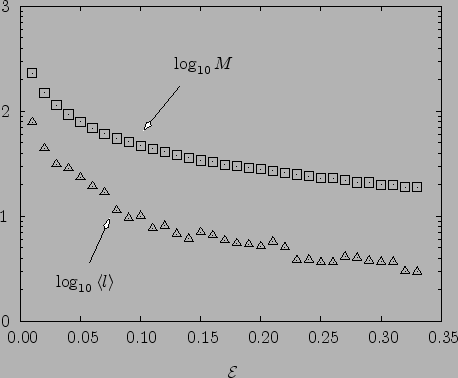Next: Graph Transformation Method Up: ENSEMBLES OF REARRANGEMENT PATHWAYS Previous: Chain Graphs   Contents
Semen A Trygubenko 2006-04-10